Sorry, you do not have permission to ask a question, You must login to ask question. Become VIP Member

Do you need to remove the ads? Become VIP Member

## What is a Curve in Roads?

A curve is nothing but an arc which connects two straight lines which are separated by some angle called deflection angle. This situation occurs where the alignment of a road way or rail way changes its direction because of unavoidable objects or conditions. The object may be a hill or a lake or a temple etc. so, for the ease of movement of vehicle at this point a curve is provided.## Types of Curves in Alignment of Highways

In general, there are two types of curves and they are

• Horizontal curves
• Vertical curves

### Horizontal Curves

The curve provided in the horizontal plane of earth is called as horizontal curve. In connects two straight lines which are in same level but having different directions. Horizontal curves are of different types as follows

• Simple circular curve
• Compound curve
• Reverse curve
• Transition curve
• Spiral
• Lemniscate

#### Simple Circular Curve

Simple circular curve is normal horizontal curve which connect two straight lines with constant radius.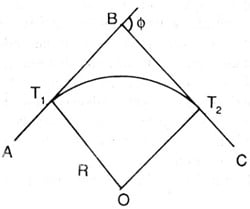#### Compound Curve

Compound curve is a combination of two or more simple circular curves with different radii. In this case both or all the curves lie on the same side of the common tangent.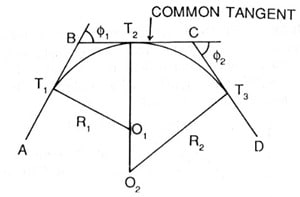#### Reverse Curve

Reverse curve is formed when two simple circular curves bending in opposite directions are meet at a point. This points is called as point of reverse curvature. The center of both the curves lie on the opposite sides of the common tangent. The radii of both the curves may be same or different.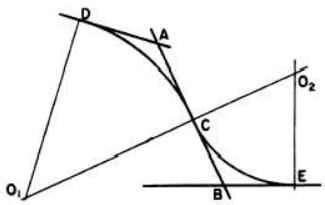#### Transition Curve

A curve of variable radius is termed as transition curve. It is generally provided on the sides of circular curve or between the tangent and circular curve and between two curves of compound curve or reverse curve etc. Its radius varies from infinity to the radius of provided for the circular curve.

Transition curve helps gradual introduction of centrifugal force by gradual super elevation which provides comfort for the passengers in the vehicle without sudden jerking.#### Spiral Curve

Spiral is a type of transition curve which is recommended by IRC as ideal transition curve because of its smooth introduction of centrifugal acceleration. It is also known as clothoid.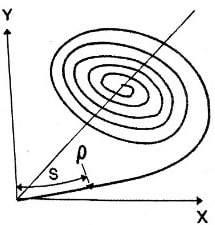#### Lemniscate

Lemniscate is a type of transition curve which is used when the deflection angle is very large. In lemniscate the radius of curve is more if the length of chord is less.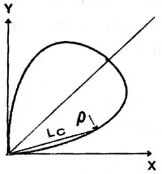### Vertical Curves

The curves provided in vertical plane of earth is called as vertical curve. This type of curves are provided when the ground is non-uniform or contains different levels at different points. In general parabolic curve is preferred as vertical curve in the vertical alignment of roadway for the ease of movement of vehicles. But based on the convexity of curve vertical curves are divided into two types

• Valley curve
• Summit curve

#### Valley Curve

Valley curve connects falling gradient with rising gradient so, in this case convexity of curve is generally downwards. It is also called as sag curve.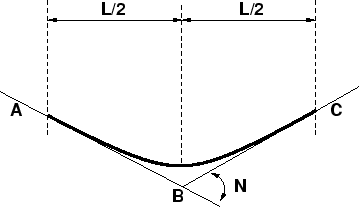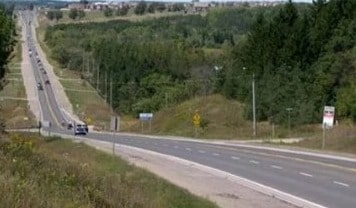#### Summit Curve

Summit curve connects rising gradient with falling gradient hence, the curve has its convexity upwards. It is also called as crest curve.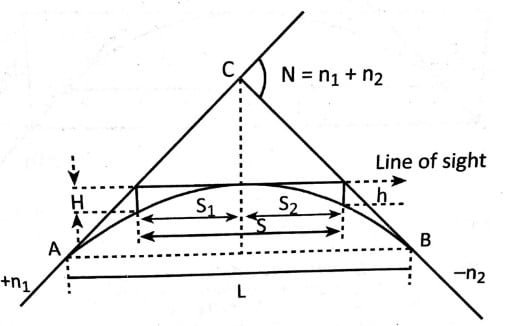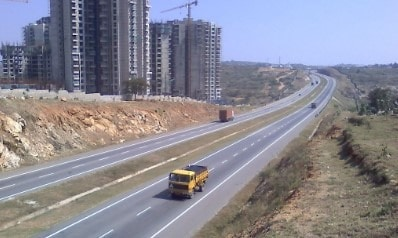Horizontal Transition Curves for Highways and Its Calculation

What is Vertical Alignment of Highways? Gradients and Vertical Curves